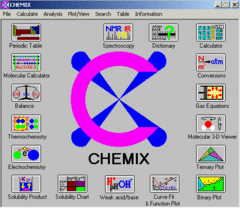Search
SoftwareCHEMIX School is an educational tool for learning chemistry. It is geared toward college-level chemistry, but is also appropriate for high-school students, chemists, and teachers. It is equipped with a periodic table containing history, 19 physical properties + number of stable isotopes, abundance, atomic mass, spin, resonance frequencies, relative receptivity, magnetic moment, quadropole moment and magnetogyric ratio for all stable isotopes. Also included are physical properties of more than 2500 unstable isotopes such as: atomic mass, half-life, decay modes, decay energy, particle energy, particle intensities,spin and magnetic moment including decay trees for 600 decays. It is also equipped with a molecular 3-D viewer, calculator, curve fit, function plot, data manipulation, derivatives, definite integrals for one and two functions, ternary phase diagram plotter, binary phase diagram plotter, trend plots of physical/chemical properties of the elements, spline interpolation, conversion table (temperature, pressure, energy, power, length and mass), solubility chart for inorganic compounds used in inorganic analysis, dictionary, and advanced calculators for molecules (Mol, mass and mass%), thermochemistry , electrochemistry, weak acid/base/buffers, acid-base indicators table, solubility (Ksp) and common ion effect calculator, gas equations, spectroscopy assignment tool (NMR H C, IR and MS) and stoichiometry (chemical equation balancer that also balances chemical equations containing free electrons). Ability to insert colors and angled text in graphics. Hi resolution print and copy to clipboard. Chemistry lessons and problems are included. Both English and Norwegian versions are available